# 基于S变换的特征信号分析 Characteristic Signal Analysis Based on S-Transform

DOI: 10.12677/APP.2019.93018, PDF, HTML, XML, 下载: 537  浏览: 2,469  科研立项经费支持

Abstract: At present, the problem of mine safety in China is becoming more and more prominent. Mine mining is accompanied by rock rupture. The time frequency analysis is used to study the multi-source signals of rock rupture. Commonly used time-frequency analysis methods include short-time Fourier transform, wavelet transform, and S transform. This paper first expounds the principle of various time-frequency analysis methods and compares and selects them to know that S transformation is the optimal time-frequency analysis method for processing various signals. Through the S transformation, signals such as rock rupture, blasting, percussion, drilling, forklift, etc. are processed to classify the outstanding characteristics of the signal, such as frequency, energy, amplitude, duration, etc., making the signal easy to distinguish. It provides some help for feature extraction and analysis of multi-source signals.

1. 引言

2. 多源信号的提取方法综述

2.1. 时频分析方法

2.1.1. 短时傅里叶变换

$h\left(f\right)={{\sum }_{+\infty }^{-\infty }h\left(t\right)e}^{-i2\text{π}ft}dt$ (1)

Fourier变换是将信号时域映射到频率域，整体上，使信号丢失了时域特性，在处理信号过程中，加入窗函数g(t)，并对非平稳信号h(t)截选，将小段信号转换到频率域通过Fourier变换，随着时间轴上窗函数的移动，每部分信号都被过渡到了频率域，同时也含有了时域特征，最终汇成整段信号的时频分布结果，这种方法将时域信号转换成时频信号，是信号分析领域的重大突破性研究，其中，时窗函数的数学表达式为   ：

${g}_{\tau ,\omega }\left(t\right)=g\left(t-\tau \right){e}^{-i\omega t}$ (2)

$STFT\left(\tau ,\omega \right)={\int }_{-\infty }^{+\infty }h\left(t\right)g\left(t-\tau \right){e}^{-i\omega t}dt$ (3)

$STFT\left(\tau ,\omega \right)$ 是h(t)的短时傅里叶变换结果，并需要满足以下能量归一化条件的要求，即

$‖g\left(t\right)‖={{\int }_{-\infty }^{+\infty }|g\left(t\right)|}^{2}dt=1,‖{g}_{\tau ,\omega }‖={{\int }_{-\infty }^{+\infty }|{g}_{\tau ,\omega }|}^{2}dt=1$ (4)

2.1.2. 小波变换

$CWT\left(a,b\right)=\frac{1}{\sqrt{a}}{\int }_{-\infty }^{+\infty }h\left(t\right)\psi \left(\frac{t-b}{a}\right)dt$ (5)

2.1.3. S变换

S变换最早是由Stockwell于1996年提出  ，依照短时傅里叶变换公式(3)，加入带有频率变量窗函数进而推出S变换公式，短时傅里叶变换依靠时窗函数截取信号从使局部分析的功能实现，短时傅里叶变换的处理精度受窗函数选取的直接影响，又因为采用Gauss窗函数可以获得信号最小时宽与带宽乘积  ，因此定义Gauss窗函数为：

$g\left(t\right)=\frac{1}{2\text{π}}{e}^{-\frac{{t}^{2}}{2}}$ (6)

$g\left(t,\sigma ,\tau \right)=\frac{1}{\sigma \sqrt{2\text{π}}}{e}^{-\frac{{\left(t-\tau \right)}^{2}}{2{\sigma }^{2}}}$ (7)

$S\left(\tau ,f,\sigma \right)={\int }_{-\infty }^{+\infty }h\left(t\right)\frac{1}{\sigma \sqrt{2\text{π}}}{e}^{\frac{-{\left(t-\tau \right)}^{2}}{2{\sigma }^{2}}}{e}^{-2\text{π}fti}dt$ (8)

$\sigma \left(f\right)=\frac{1}{|f|}$ (9)

$S\left(\tau ,f\right)={\int }_{-\infty }^{+\infty }h\left(t\right)\frac{|f|}{\sqrt{2\text{π}}}{e}^{\frac{-{f}^{2}{\left(t-\tau \right)}^{2}}{2}}{e}^{-2\text{π}fti}dt$ (10)

$\phi \left(t,f\right)=\frac{|f|}{\sqrt{2\text{π}}}{e}^{\frac{-{f}^{2}{\left(t-\tau \right)}^{2}}{2}}{e}^{-2\text{π}fti}$ (11)

3. 时频分析方法选择

3.1. 时频变换比较

S变换  的公式既可由短时傅里叶变换推出又可由小波变换推出，S变换是前两种方法的继承与发展。S变换法与短时傅里叶变换法相比的最大优点在，其高斯窗函数中的频率变量可以根据具体的频率大小来调节从而实现对时频分辨率的控制；与小波变换相比的最大优势在于，它可以反映出信号真正的时间频率谱，完整地描述信号特征，另外S变换拥有短时傅里叶变换和小波变换的线性特征，对于多分量的合成信号来说，S变换结果不存在交叉项，使其时频分析成效得到了大幅提升  。

3.2. S变换的优势

4. 特征信号图谱以及提取

4.1. 特征信号图谱分析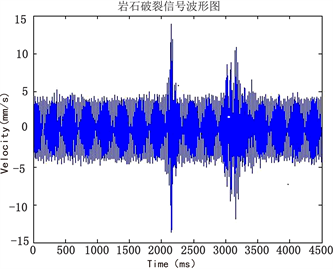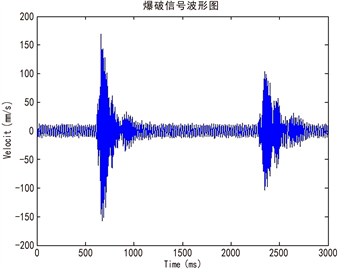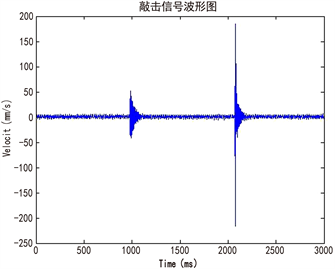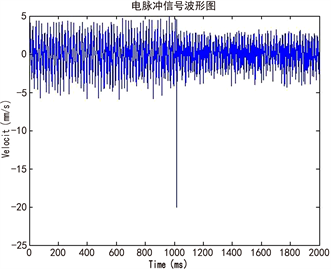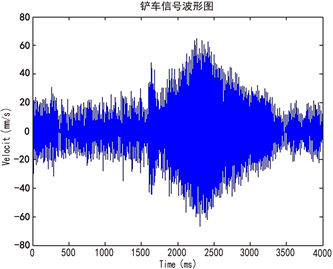Figure 1. The original atlas of signals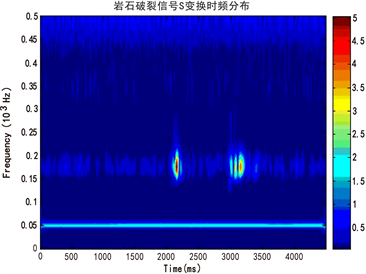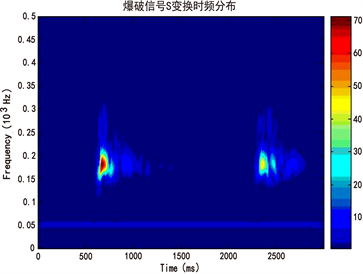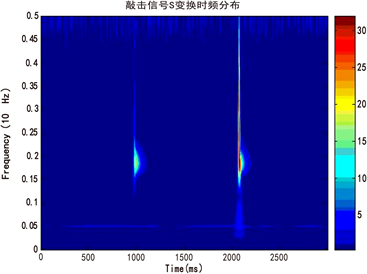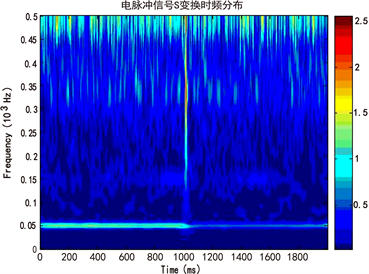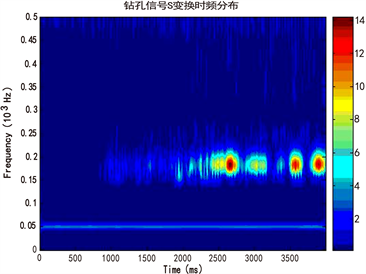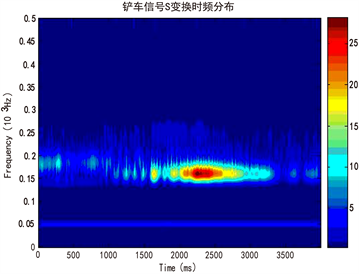Figure 2. Time-frequency spectrum of S-transform signal

4.2. 特征信号分析Table 1. Characteristic range of various vibration signals

5. 结论

NOTES

*通讯作者。

  康富. 新时期地质矿产勘查找矿技术分析[J/OL]. 世界有色金属, 2018(20): 81-82.  赵向东, 王育平, 陈波, 姜福兴. 微地震研究及在深部采动围岩监测中的应用[J]. 合肥工业大学学报(自然科学版), 2003(3): 363-367.  黄昱丞, 郑晓东, 栾奕, 杨廷强. 地震信号线性与非线性时频分析方法对比[J]. 石油地球物理勘探, 2018, 53(5): 975-989+882.  李恒, 张氢, 秦仙蓉, 孙远韬. 基于短时傅里叶变换和卷积神经网络的轴承故障诊断方法[J]. 振动与冲击, 2018, 37(19): 124-131.  Stein, E.M. (2006) Fourier Analysis: An Introduction. Princeton University Press, Princeton, 96-121.  杨巧荣. 微地震监测技术在泸沽铁矿中的应用与分析[D]: [硕士学位论文]. 绵阳: 西南科技大学, 2014.  Mansinha, I., Stockwell, R.G. and Lowe, R.P. (1997) Pattern Analysis with Two-Dimensional Spectral Localisation: Applications of Two-Dimensional S Transforms. Physica, 239, 286-295. https://doi.org/10.1016/S0378-4371(96)00487-6  陈学华. 时频分布与地震信号谱分析研究[D]: [硕士学位论文]. 成都: 成都理工大学, 2006.  武国宁, 曹思远, 马宁, 等. S变换的时频分析特性及其改进[J]. 地球物理学进展, 2011, 26(5): 1661-1667.  周安. 时频分析在地震资料处理中的应用[D]: [硕士学位论文]. 长沙: 中南大学, 2010.  Stockwell, R.G., Mansinha, L. and Lowe, R.P. (1996) Localization of the Complex Spectrum: The S Transform. IEEE Transactions on Signal Processing, 44, 998-1001. https://doi.org/10.1109/78.492555  冯国勇. 基于小波变换的金属表面缺陷检测算法[J]. 世界有色金属, 2018(20): 231-233.  Rajeev Ranjan, A.K. and Singh, N.J. (2018) Formulation of Some Useful Theorems for S-Transform. Optik, 168, 913-919.  任煦. 金川镍矿微地震弱信号提取及分析[D]: [硕士学位论文]. 绵阳市: 西南科技大学, 2016.  单娜琳, 程志平, 丁彦礼. 地震映像数据的时频分析方法及应用[J]. 地球物理学进展, 2007, 22(6): 1740-1745.